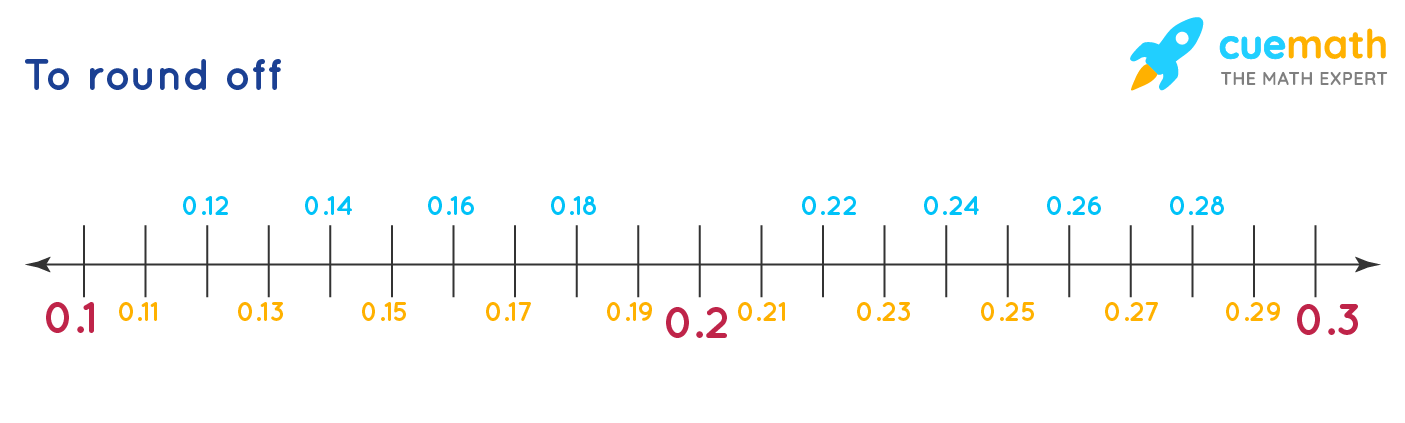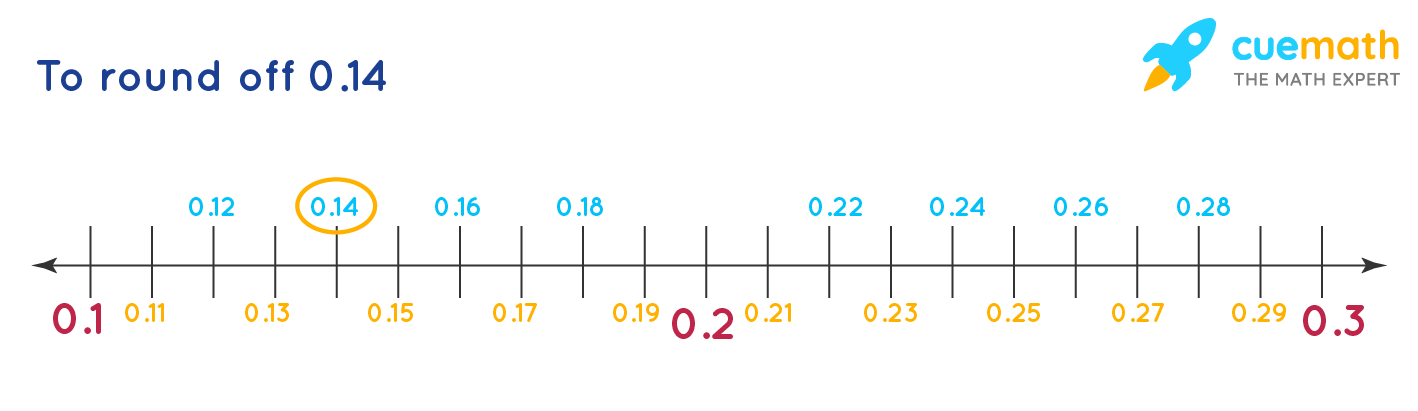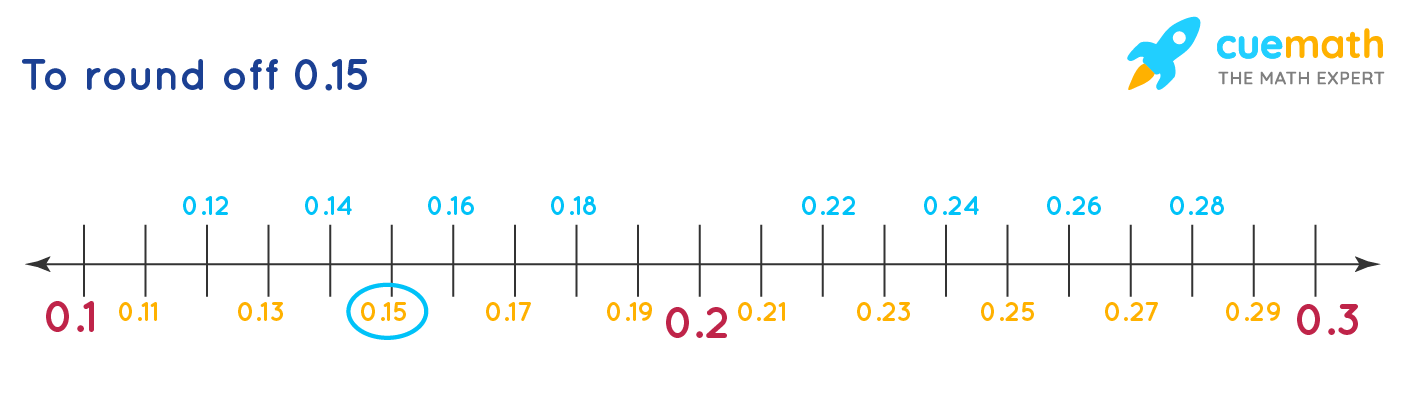# What Does it Mean to Round to the Nearest Tenth?

## Question: What does it mean to round to the nearest tenth?

Rounding off a number is done to get the more accurate result

## Answer: Rounding off a number to the nearest tenth means that you have to look or find the tenth which is closest to the given number and then write that as the rounded off number

Lets look at this number line to understand this:

## Explanation:### Case 1: To round off 0.14The nearest tenth to 0.14 is 0.1. Therefore 0.14 can be rounded off to 0.1.

### Case 2: To round off 0.15The number 0.15 is exactly in the middle of 0.1 and 0.2, but we will always take the higher tenth in such case. Therefore 0.15 can be rounded off to 0.2